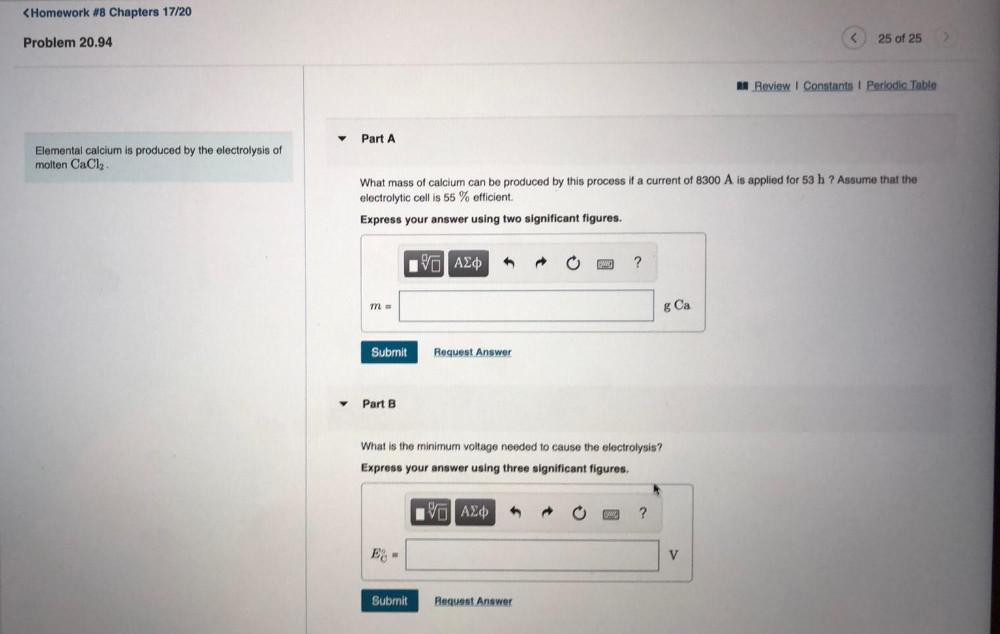Question:

# Elemental calcium is produced by the electrolysis of molten

Last updated: 7/28/2022Elemental calcium is produced by the electrolysis of molten CaCl₂. 1. What mass of calcium can be produced by this process if a current of 8300 A is applied for 53 h? Assume that the electrolytic cell is 55 % efficient. Express your answer using two significant figures. 2. What is the minimum voltage needed to cause the electrolysis? Express your answer using three significant figures.